# Introduction

I tried solving `"the maximum average section of daily visitor numbers (equivalent to paiza rank B)"` from the skill check past problem collection (Java): blush: I am a beginner in programming, but I also tried to summarize the algorithm part in an easy-to-understand manner! By the way, the author is B rank who has no practical experience. ..

• Problem
• How to solve

# Problem

### Problem statement

You managed a website. I have run a campaign on this website for k consecutive days, but I forgot when and how long.

Fortunately, we have access logs for all n days running the website, and we know the number of visitors per day. For the time being, I decided to consider the period with the highest average number of visitors per day for the consecutive k days as a candidate for the period of the campaign.

Since the list of visitor number for n days and the number of days k of the campaign are entered, output the number of candidates for the period of the campaign and the earliest start date among the candidates.

### Value entered

The input consists of two lines. In the first line, n and k are entered separated by a space. In the 2nd line, n integers a_1, a_2, …, a_n are entered separated by half-width spaces. a_i represents the number of visitors on day i. ***

### Expected output

Please output the number of candidates for the campaign period and the earliest start date among the candidates in this order in one line separated by a space. ***

### Condition

The following conditions are satisfied in all test cases. ・1≦n≦1,000 ・1≦k≦n ・0≦a_i≦100 ***

#### ` Input example 1`

```text

5 3 1 2 3 2 1 ```

```text

1 2 ```

#### ` Input example 2`

```text

10 2 6 2 0 7 1 3 5 3 2 6 ```

#### ` Output example 2`

```text

5 1 ```

Please check the problem from here for details!

# How to solve

### 1. Think about the general flow

I usually write the code after commenting out the general flow. This time I tried to draw it for easy understanding!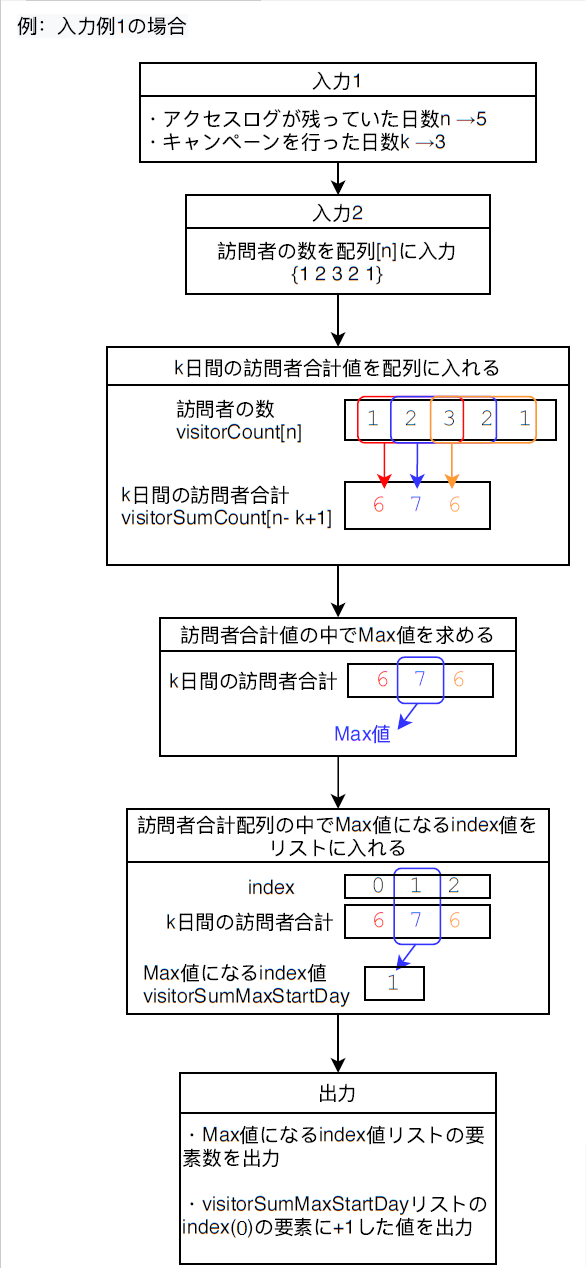### 2. Try writing the code

I will write the code considering the rough flow. I will write the code along each process. Where the scope is likely to be long, it is divided into methods.

#### ① Input 1

Standard input uses Scanner class.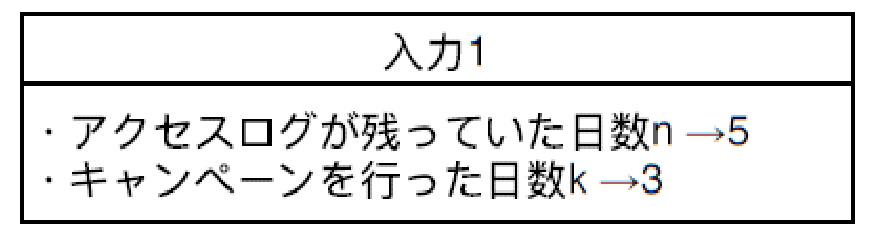``````// input
Scanner sc = new Scanner(System.in);
// number of days that the access log was left n
int n = sc.nextInt();
// Number of days the campaign was held k
int k = sc.nextInt();
``````

#### ② Input 2Divide into methods. First, write the initialization of the array and the method call. ↓

``````// n days visitor array
int[] visitorCount = new int[n];
// Enter the number of visitors (n days)
visitorCount = inputVisitor(n, visitorCount, sc);
``````

Next, write the contents of the method. ↓

``````/**
* Method to enter the number of visitors (for n days)
* @param n The number of days that the access log was left n
* @param visitorCount Array of visitor count for n days
* @param sc standard input
* @return Array with the number of visitors
*/
private static int[] inputVisitor(int n, int[] visitorCount, Scanner sc) {
for(int i = 0; i <n; i++) {
visitorCount[i] = sc.nextInt();
}
return visitorCount;
}
``````

#### ③ Make an array of total visitor values for k days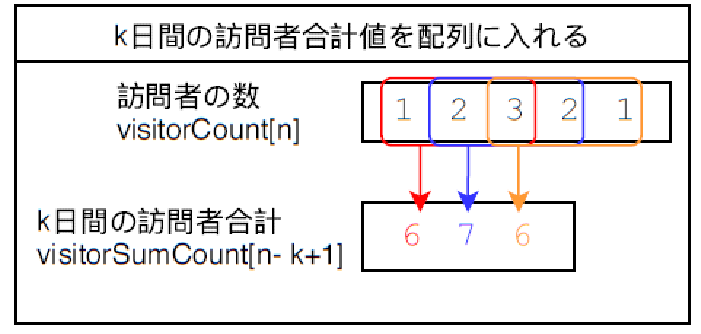Divide into methods. First, write the initialization of the array and the method call. ↓

``````// Array of total number of visitors for k days [n-k + 1]
int[] visitorSumCount = new int[n-k + 1];
// Calculate visitorSumCount
visitorSumCount = visitorSum(visitorCount, n, k, visitorSumCount);
``````

Next, write the contents of the method. ↓

``````/**
* Find the total number of visitors for k days ((n-k + 1) times)
* @param visitorCount Array of visitor count for n days
* @param n The number of days that the access log was left n
* @param k The number of days the campaign was conducted k
* @param visitorSumCount Array of total number of visitors for k days
* @return An array that calculates the total number of visitors for k days
*/
private static int[] visitorSum(int[] visitorCount, int n, int k, int[] visitorSumCount) {
for(int i = 0; i <(n-k + 1); i++) {
for(int j = i; j <(k + i); j++) {
visitorSumCount[i] += visitorCount[j];
}
}
return visitorSumCount;
}
``````

#### ④ Calculate the maximum value among the total visitor values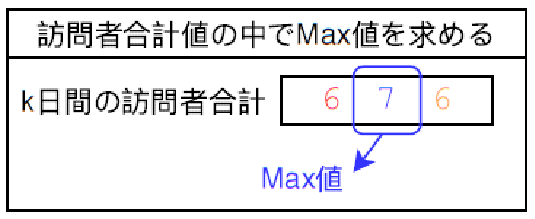Divide into methods. First, write variable initialization and method invocation. ↓

``````// Max value in visitorSumCount
int visitorSumMax = 0;
// seek visitorSumMax
visitorSumMax = sortVisitorSumMax(visitorSumCount, visitorSumMax);
``````

Next, write the contents of the method. ↓

``````/**
* Calculate the maximum value among the total visitor values
* @param visitorSumCount Array of total number of visitors for k days
* @param visitorSumMax Max value in visitorSumCount
* @return Max value obtained
*/
private static int sortVisitorSumMax(int[] visitorSumCount, int visitorSumMax) {
for(int i = 0; i <visorSumCount.length; i++) {
if(visitorSumCount[i]> visitorSumMax) {
visitorSumMax = visitorSumCount[i];
}
}
return visitorSumMax;
}
``````

#### ⑤ Add the index value that becomes the Max value in the visitor total array to the list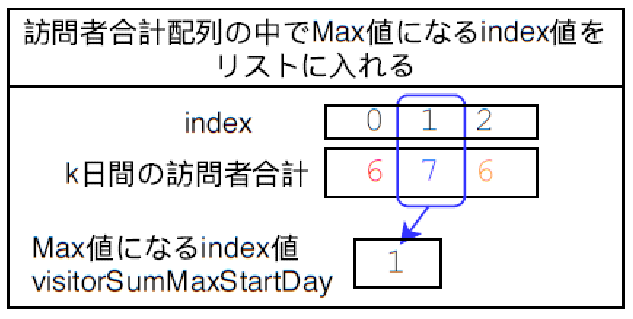``````// A list containing index values that will be visitorSumMax
List<Integer> visitorSumMaxStartDay = new ArrayList<>();
// Request visitorSumMaxStartDay
visitorSumMaxStartDay = visitorSumMaxStartDaySort(visitorSumCount, visitorSumMax, visitorSumMaxStartDay);
``````

Next, write the contents of the method. ↓

``````/**
* Save the index that becomes visitorSumMax in visitorSumCount to the list.
* @param visitorSumCount Array of total number of visitors for k days
* @param visitorSumMax Max value in visitorSumCount
* @param visitorSumMaxStartDay Index of visitorSumMax in visitorSumCount
* @return returns a list with indexes
*/private static List<Integer> visitorSumMaxStartDaySort(int[] visitorSumCount, int visitorSumMax, List<Integer> visitorSumMaxStartDay) {
for(int i = 0; i <visorSumCount.length; i++) {
if(visitorSumCount[i] == visitorSumMax) {
}
}
return visitorSumMaxStartDay;
}
``````

#### ⑥ Output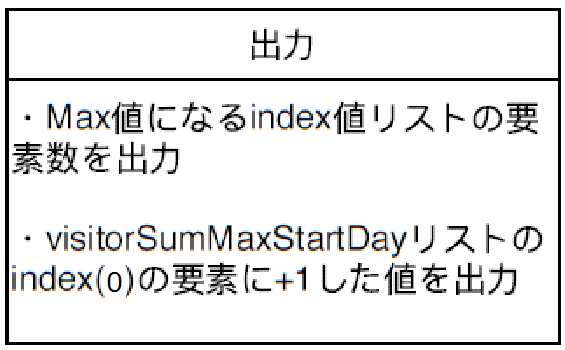This is the last! visitorSumMaxStartDay Outputs the number of elements in the list and the value of index (0) + 1.

``````// output the number of candidate days
System.out.print(visitorSumMaxStartDay.size() + "");
// output the first day of the candidate date
System.out.print(visitorSumMaxStartDay.get(0) + 1);
``````

``````import java.util.*;

public class PaizaB01Visitors {

public static void main(String[] args) throws Exception {
Scanner sc = new Scanner(System.in);
// number of days that the access log was left n
int n = sc.nextInt();
// Number of days the campaign was held k
int k = sc.nextInt();

// n days visitor array
int[] visitorCount = new int[n];
// Enter the number of visitors (n days)
visitorCount = inputVisitor(n, visitorCount, sc);

// Array of total number of visitors for k days [n-k + 1]
int[] visitorSumCount = new int[n-k + 1];
// Calculate visitorSumCount
visitorSumCount = visitorSum(visitorCount, n, k, visitorSumCount);

// Max value in visitorSumCount
int visitorSumMax = 0;
// seek visitorSumMax
visitorSumMax = sortVisitorSumMax(visitorSumCount, visitorSumMax);

// A list containing index values that will be visitorSumMax
List<Integer> visitorSumMaxStartDay = new ArrayList<>();
// Request visitorSumMaxStartDay
visitorSumMaxStartDay = visitorSumMaxStartDaySort(visitorSumCount, visitorSumMax, visitorSumMaxStartDay);

// output the number of candidate days
System.out.print(visitorSumMaxStartDay.size() + "");
// output the first day of the candidate date
System.out.print(visitorSumMaxStartDay.get(0) + 1);

sc.close();
}

/**
* Method to enter the number of visitors (for n days)
* @param n The number of days that the access log was left n
* @param visitorCount Array of visitor count for n days
* @param sc standard input
* @return Array with the number of visitors
*/
private static int[] inputVisitor(int n, int[] visitorCount, Scanner sc) {
for(int i = 0; i <n; i++) {
visitorCount[i] = sc.nextInt();
}
return visitorCount;
}

/**
* Find the total number of visitors for k days ((n-k + 1) times)
* @param visitorCount Array of visitor count for n days
* @param n The number of days that the access log was left n
* @param k The number of days the campaign was conducted k
* @param visitorSumCount Array of total number of visitors for k days
* @return An array that calculates the total number of visitors for k days
*/
private static int[] visitorSum(int[] visitorCount, int n, int k, int[] visitorSumCount) {
for(int i = 0; i <(n-k + 1); i++) {
for(int j = i; j <(k + i); j++) {
visitorSumCount[i] += visitorCount[j];
}
}
return visitorSumCount;
}

/**
* Calculate the maximum value among the total visitor values
* @param visitorSumCount Array of total number of visitors for k days
* @param visitorSumMax Max value in visitorSumCount
* @return Max value obtained
*/
private static int sortVisitorSumMax(int[] visitorSumCount, int visitorSumMax) {
for(int i = 0; i <visorSumCount.length; i++) {
if(visitorSumCount[i]> visitorSumMax) {
visitorSumMax = visitorSumCount[i];
}
}
return visitorSumMax;
}

/**
* Save the index that becomes visitorSumMax in visitorSumCount to the list.
* @param visitorSumCount Array of total number of visitors for k days
* @param visitorSumMax Max value in visitorSumCount
* @param visitorSumMaxStartDay Index of visitorSumMax in visitorSumCount
* @return returns a list with indexes
*/
private static List<Integer> visitorSumMaxStartDaySort(int[] visitorSumCount, int visitorSumMax, List<Integer> visitorSumMaxStartDay) {
for(int i = 0; i <visorSumCount.length; i++) {
if(visitorSumCount[i] == visitorSumMax) {
}
}
return visitorSumMaxStartDay;
}
}
``````

# in conclusion

Thank you for watching this! If you have any comments or points for improvement, we would appreciate it if you could comment.

Tags:

Updated: